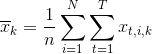# Sequence-Wise Batch-Norm

I have a question regarding sequence-wise batch-normalization for RNNs, as described in the paper: https://arxiv.org/abs/1510.01378[Note: I swapped the indices in the formula to be consistent with sequence-first format]

Assuming variable length sequences, what would be the best way to implement this as a layer in PyTorch?

My first idea was to manually compute the inner sum (i.e. along the time axis for each sequence independently), create a new vector of size `(N, H)` where `N` is the mini-batch size and `H` the number of features, with this vector containing the sum of all outputs for each sequence, and then call `BatchNorm1d` on that.

However, this will not work properly for the standard deviation (and probably for other things as well).

Has anyone implemented this already in PyTorch? What is the best way to do this?